Applied and Computational Mathematics
Volume 4, Issue 3, June 2015, Pages: 116-121

Study on Dynamic Risk Measurement Based on ARMA-GJR-AL Model

Hong Zhang, Li Zhou, Jian Guo

School of Information, Beijing Wuzi University, Beijing, China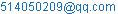(Hong Zhang)

Hong Zhang, Li Zhou, Jian Guo. Study on Dynamic Risk Measurement Based on ARMA-GJR-AL Model. Applied and Computational Mathematics. Vol. 4, No. 3, 2015, pp. 116-121. doi: 10.11648/j.acm.20150403.13

Abstract: This paper established the ARMA-GJR-AL model of dynamic risk VaR and CVaR measurement. Considering from aspects of the correlation and volatility and residual distribution characteristics, studying the dynamic risk measures of VaR and CVaR based on ARMA-GJR-AL model. Through empirical research, Risk prediction and accuracy of inspection are given of the Shanghai stock market and the New York stock market. And we study the effectiveness of the model. The results show that the dynamic risk measurement model based on AL distribution is more reasonable and applicability, so it can effectively measure risk.

Keywords: ARMA-GJR-AL Model, VaR, Financial Market Risk

1. Introduction

The distribution of risk in financial markets not only on the edge always has a significant peak and the fat tail, and features such as asymmetrical, but also often shows self correlation, heteroscedasticity and leverage effect phenomenon. In order to accurately measure the VaR and the CVaR, hoping to capture these characteristics in a certain extent, ARMA model (Box et al., 1994) and GARCH model (Bollerslev, 1986) have been widely applied.

Bollerslev (1986)3-6 on the basis of in-depth study on the ARCH model, extend the model to a more general infinite error term. And then introduce the pre conditional variance in regression in analysis, research and propose the GARCH model (or generalized ARCH model), which makes the model identification, parameter estimation and the establishment and are more convenient. Engle, Lilien and Robbins (1987)7-10 joined the analysis of the risk premium in the research, put forward the ARCH-M model and GARCH-M model, which makes the study linked the conditional variance and the conditional mean, provide a new method for estimating and testing the time-dependent risk compensation. In the Black (1976)1-2 study, shows that the impact of good news and bad news on market market is not the same, Then, some non symmetric GARCH model was proposed to describe the study of the market risk characteristics. Zakoian (1990)15 proposed the TARCH model, Virtual variables used in the study to reflect the good or bad news of different impact on market volatility. Nelson (1990, 1991)12-14 studied EGARCH model (Exponential GARCH),and describe the leverage effect of market volatility using the logarithmic form in the variance model. Glosten et al. (1993)11 on the base of previous study  proposed Heteroscedastic Model of non symmetrical, referred to as the GJR model, This model not only has advantages of less general GARCH model that fewer parameters estimated and can well describe the volatility asymmetry. Due to the importance of volatility and risk in the financial analysis, Research on GARCH model of the front is widely used in many aspects of financial time series modeling, market risk measurement and management etc.

There are 3 Levels titles in an article to make ideas clear:

(1)Given the establishment of ARMA (1,1)-GJR(1.1)-AL model

(2)Given the prediction and test about VaR and CVaR

(3)Given the comprehensive analysis about the model

2. The Empirical Analysis

2.1. The Selection of Data and Its Characteristics

Selecting S.H.I (Shanghai composite index) and N.Y. composite index as research objects. Sample interval is from 2010.01.04 to 2014.12.31.Using Logarithm yields,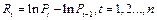The results of statistics (table 1) show that tail of exponential gains and losses distribution is fatter than normal distribution’s. which mains abnormal fluctuations in the market happen sometimes, The fact that skewness are all negative shows , from a long-term perspective, that fluctuation in the left side of exponential gains and losses distribution is larger than right side. So normal distribution cannot effectively characterize these phenomena.  Stationary ADF-test results that H=1and P=1.0e-0.3 are far less than 0.05, showing the results reject unit root process hypothesis, and accept the hypothesis of stationary sequence.

Quantitative study shows the distribution with the correlation and the ARCH phenomenon, testing its lag value(101520) by Ljung-Bo-Q and Engle’s ARCH. In the 5% significant level.(table 2)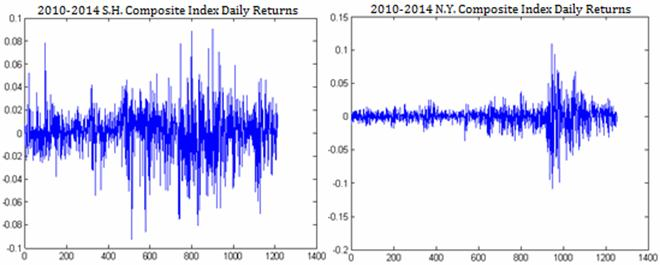Figure 1. The return series of Shanghai Composite Index (left) and the New York composite index (right).

Table 1. Yield-related statistics and its stationarity test results.

 Yield Mean Variance STD Slewness Kurtosis ADF-test S.H. 0.000 0.000 0.020 -0.352 5.454 1(0.001) N.Y. 0.000 0.000 0.014 -0.681 12.547 1(0.001)

Notes :data is the H value of backtesting ;( Parentheses are the P value )

Table 2. Yield-related statistics and its ARCH test results.

 Lag order S.H. N.Y. Correlation test Square serial correlation test ARCH text Correlation test Square serial correlation test ARCH text 10 0(0.201) 1(0) 1(0.000) 1(0.000) 1(0) 1(0) 15 1(0.003) 1(0) 1(0.000) 1(0.000) 1(0) 1(0) 20 1(0.004) 1(0) 1(0.000) 1(0.000) 1(0) 1(0)

Notes: data is the H value of backtesting ;( Parentheses are the P value)

2.2. The Establishment of Arma(1,1)-Gjr(1.1)-Al Model

2.2.1. Parameter Estimation

We capture the characteristics of stock market risk using ARMA (1,1)-GJR(1,1)-AL model. Modeling on the observed sample: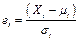(1)

and getting the AL(e) distribution series whose standardized residual series is I.I.D.

Known from the theory of AL distribution, the mean and variance of the standard error are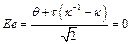(2)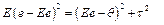(3)

So the parameter of the AL distribution: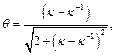(4)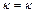(5)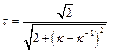(6)

Known from above, the actual estimated parameters are underestimated. Now, we estimate the parameters of ARMA (1,1)-GJR(1,1)-AL by the maximum likelihood estimation. Its Joint probability density function is: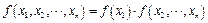(7)

And its Logarithmic likelihood function is: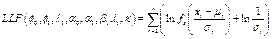(8)

With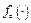is the density distribution function. Through nonlinear optimization, Estimating the parameters of the model using MATLAB.(table 3),then get the parameter estimation of the AL(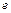) distribution.(table 4).At the same time, given the parameter estimation of ARMA(1,1)-GJR(1,1)-N.

Known from the table 3, the log likelihood function values of two models are both great the main parameters are significantly, but individual constants and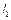. This means that they have successfully described the volatility. And they could be used as a powerful  tool to analyze stock fluctuation behavior; As you can see from the result of parameter estimation of ARMA(1,1)-GJR(1,1)-AL,  There are obvious heteroscedasticity and degree of leverage effect of Shanghai Composite Index and New York composite index. Parameters L1 are all positive suggests that stock returns show different response to the same degree of negative and positive impact. (the bad news caused yields fell  is  more than the  yield increases caused by the same degree of good news ).

Meanwhile, the main parameter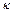of AL(e) distribution are greater than 1. Showing that yield distributions of the Shanghai Composite Index and the New York Composite Index both have asymmetry and fat tails.

The fact that the test results (J-B and K-S) of the normal distribution of the two models in terms of standard residuals ,in the 5% or 1% significant level ,accept the estimated AL(e) distribution hypothesis, and reject the hypothesis of normal distribution.(table 4) And known from two index’s of fitting map of  AL(e )distribution of standard residual.(figure 2) we can determine that the AL distribution assumption is more reasonable than the normal distribution.

Table 3. Parameter estimation of ARMA (1,1) -GJR (1,1) model.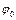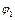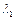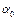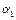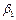LLF S.H. Normal distribution 0.003**(2.842) -0.524**(-4.253) 0.845**(5.145) 0.000**(2.954) 0.954**(103.142) 0.065**(4.256) 0.022(1.541) 3154.5 AL distribution 0.002**(3.326) -0.842**(-75.214) 0.842**(84.515) 0.000*(2.248) 0.941**(65.259) 0.047**(5.241) 0.013(0.5014) 1.021(84.254) 3125.2 N.Y. Normal distribution 0.000(0.671) 0.243(0.847) -0.419(-1.069) 0.000**(5.452) 0.947**(71.215) 0 (0) 0.159**(5.324) 3951.2 AL distribution 0.000**(2.958) 0.756**(6.484) -0.754**(-4.547) 0.000(0.214) 0.951**(8.245) 0 (0) 0.147(0.0154) 1.155**(8.951) 3965.2

Note: the brackets is t-value, * and * * respectively indicate in the 5% and 1% significant level; LLF is the log likelihood function value.

Table 4. Estimation of the parameters of AL distribution and residual distribution.

 Standardized residuals of S.H. Standardized residuals of N.Y. AL distribution parameters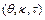=(0.136,1.102,0.996) AL distribution parameters=(0.236,1.152,0.986) Normal distribution J-B text AL distribution K-S text Normal distribution J-B text AL distribution K-S text 1(0.001) 0(0.425) 1(0.001) 0(0.142)

Note: the form data is the H value; the brackets are the P value.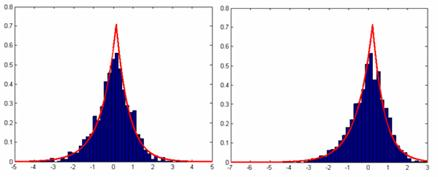Figure 2. AL distribution fitting chart of standard residuals of the Shanghai market (left) and the New York market (right).

2.2.2. The Analysis of Standardized Residuals

In order to further test the validity of the model, we analyze the standard residual error sequence of sample data after filtering. The mean of standard residual error sequence approximation is 0 and standard deviation of standard residual error sequence approximation is 1; We also could know from table 5, that Skewness is left fat tail and Kurtosis is spike. Ljung-Box-Q test and Engle’s ARCH are performed on the standardized residuals, respectively. The relevance and ARMA phenomenon are eliminated basically in the 5% significant level. ARMA(1,1)-GJR(1.1)-AL model can be considered to capture market risk features very good.

Table 5. standard residual correlation statistics and correlation test and ARCH test.

 Statistic Standardized residuals of S.H. Standardized residuals of N.Y. Mean Std Skewness Kurtosis Mean Std Skewness Kurtosis -0.010 0.960 -0.325 4.758 -0.012 0.958 -0.758 5.145 Lag orders Standardized residuals correlation test and ARCH text Standardized residuals correlation test and ARCH text correlation test Square sequence correlation test ARCH text correlation test Square sequence correlation test ARCH text 10 0(0.147) 0 (0.745) 0 (0.758) 0 (0.758) 0 (0.228) 0 (0.185) 15 1(0.042) 0 (0.842) 0 (0.846) 0 (0.547) 0 (0.526) 0 (0.458) 20 0(0.246) 0 (0.574) 0 (0.965) 0 (0.485) 0 (0.659) 0 (0.254)

Note: parentheses are P value in the significant level.

2.2.3. Prediction and Test About Var and Cvar

Based on the above analysis of the model and parameter estimates, we select the Shanghai index data for 2014 as a sample. And we assume that parameters unchanged during the prediction model. So we can calculate condition mean and variance of yield fluctuations through the model, and then calculate VaR and CVaR.

We could know from figure 3 that the CVaR estimate is much higher than that of var. It is a more conservative risk measurement tool. At the same time, in general, Shanghai stock market risk is greater than the risk of New York in the same period. Especially in the second half of 2014, The Shanghai stock market risk is more volatile.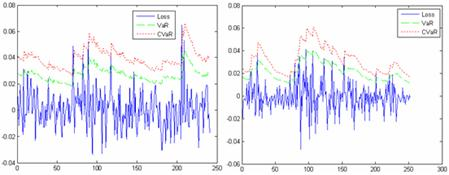Figure 3. The VaR of the Shanghai Composite Index (left) and the New York composite index (right) in the 95% level.

For the given confidence level (95%, 97.5%, 99%, 99.5%, 99.9%), the test result of the VAR and CVAR forecast is given (table 6 and table 7). At the same time, the test result of the VAR and CVAR by using ARMA(1,1)-GJR(1,1)-N model is given.

From the test results, we could sure the model with stability and applicability.

This article adopts the method of similar to the McNeil and Frey (2000) in the trading day that VAR fails. And the mean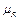and residual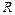can be obtained through the formula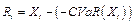(9)

A sample of 1000 sequences generated by bootstrap method to text the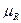(table 7).Analysis shows that when the VaR value fails, the CVaR value of the model accurately predicted the actual loss, andis closer to zero. That means CVAR more accurate estimate the tail risk. The effect of CVAR prediction (using AL method) is poorer, so it is often underestimated risk. While AL method can better predict the risk of CVaR

Table 6. VaR value test.

 Sample Risk model Test statistics 95% 97.5% 99% 99.5% 99.9% S.H ARMA-GJR-N VaR LR statistics 0.390 1.313 3.845 6.741 4.947 ARMA-GJR-AL VaR LR statistics 0.387 0.000 0.071 0.452 0.458 N.Y. ARMA-GJR-N VaR LR statistics 1.475 7.211 10.086 6.248 4.109 ARMA-GJR-AL VaR LR statistics 0.456 0.014 0.186 0.047 0.514

Note: * refused to the model.

Table 7. CVaR inspection when VaR invalid.

 Sample Measurement model R-mean 95% 97.5% 99% 99.5% 99.9% S.H ARMA-GJR-N CVaR-0.011* (-2.768) -0.004* (-1.345) -0.009 (-1.947) -0.008 (-1.487) -0.018* (-6.458) ARMA-GJR-AL CVaR-0.001 (-0.842) -0.004 (-2.487) -0.008* (-5.178) -0.255 (-1.485) # # N.Y. ARMA-GJR-N CVaR-0.006 (-3.187) -0.003* (-1.957) -0.004 (-0.784) -0.038* (-2.819) -0.005 (-5.487) ARMA-GJR-AL CVaR0.007 (1.852) 0.001 (0.754) 0.002 (1.547) 0.006 (#) # #

Note:# denotes the data does not exist; t-statistic values are shown in brackets, * and mean rejected.

3. Summary

In view of the actual financial time series and distribution characteristics of market risk, in this paper, we consider three aspects: the correlation, volatility and residual distribution. And establish models to depict the market risk characteristics. Based on the financial risk measurement tools and related theory of mathematical statistics, The risk value measurement formula based on the asymmetric Laplace distribution is given and it is concluded that the dynamic risk prediction and accuracy test.We select S.H.I and New York's composite index from 2009 to 2014 as samples to build ARMA(1,1)-GJR(1,1)-AL model and ARMA(1,1)-GJR(1,1)-N model to capture the market risk characteristics. The Shanghai stock market and the New York stock market in 2014 are calculated respectively on the day of the dynamic VaR and CVaR. The results show that the dynamic risk measurement model based on asymmetric Laplace distribution has more rationality and applicability. It can effectively predict risk.

For the stock markets are given in the paper, return series tend not to obey normal distribution. Although GJR model can describe these characteristics in a certain extent, it is often difficult to fully capture the characteristics of yield sequence that GJR model based on normal distribution assumption. Risk measurement models based on the assumption of normal distribution exist some defects, and parameter estimates may not be optimal. Asymmetric Laplace distribution can describe these characteristics well. Risk measurement models based on ARMA(1,1)-GJR(1,1)-AL distribution, whether in  the  US market or the Japanese stock market, or in  Chinese stock market which as an emerging market ,VaR or CVaR showing both a relatively good. In each of the confidence interval(95%,97.5%,99%,99.5%,99.9%) ,and  risk  measurement models based on ARMA(1,1)-GJR(1,1)-AL distribution  are more reasonable and applicable than risk  measurement models based on normal  distribution

Acknowledgements

This project (Empirical research on Stock index investment risk model, No.68) is funded by the "2014-2015 school year, Beijing Wuzi University, College students' scientific research and entrepreneurial action plan project". And by Beijing Wuzi UniversityYunhe scholars program(00610303/007). And by Beijing Wuzi UniversityManagement science and engineering Professional group of construction projects.(No.PXM2015_014214_000039)

References

1. Black F. The Dividend Puzzle [J]. Journal of Portfolio Management, 1976, 2 (2) 6-7.
2. Black F., Scholes M. The pricing of options and corporate liabilities [J]. Journal of Political Economy, 1973, 81 (3): 639-657.
3. Bollerslev T. Generalized autoregressive conditional heteroskedasticity [J]. Journal of Econometrics, 1986, 31: 317-324.
4. Bollerslev T. Generalized autoregressive conditional heteroskedasticity [J]. Journal of Econometrics, 1986, 31 (3): 309-317.
5. Bollerslev T. Modelling the Coherence in Short-Run Nominal Exchange Rates: A Multivariate Generalized ARCH Mode [J]. Review of Economics and Statistics,1990, 72: 499-503.
6. Bollerslev T., Engle R.F., Wooldridge M.J. A capital Asset Pricing Model with time-varying covariances [J]. Journal of Political Economy, 1988, 96: 119-130.
7. Engle R.F. Autoregressive conditional heteroskedasticity with estimates of the variance of United Kingdom inflation [J]. Econometric, 1982, 50 (4): 989-1004.
8. Engle R.F., Kroner F.K. Multivariate Simultaneous Generalized ARCH [J].Econometric Theory, 1995, 11: 135-149.
9. Engle R.F., Lilien D.M., Robins R.P. Estimating time-varying risk Premia in the term structure: The ARCH-M model [J]. Econometrica, 1987, 55: 395-406.
10. Engle Robert F. Dynamic Conditional Correlation: A Simple Class of Multivariate GARCH Models [J]. Journal of Business and Economic Statistics, 2002, 20 (3):341-347.
11. Glosten L. R., Jagannathan R. and Runkle D. E. On the relation between expected value and the volatility of the nominal excess return on stocks [J]. The Journal of Finance, 1993, 48 (5): 1779-1801.
12. Nelsen R.B. An introduction to Copulas [M]. New York: Springer-Verlag, 1999.
13. Nelson B. Conditional heteroscedasticity in asset returns: a new approach [J]. Econometrica, 1991, 59: 349-360.
14. Nelson D.B. ARCH models as diffusion approximations [J]. Journal of Econometrics, 1990, 45: 9-28.
15. Zakoian J.M. Threshold heteroskedastic models [J]. Journal of Economic Dynamics and Control, 1990, 18: 937-945.

 Contents 1. 2. 2.1. 2.2. 3.
Article ToolsAbstractPDF(265K)